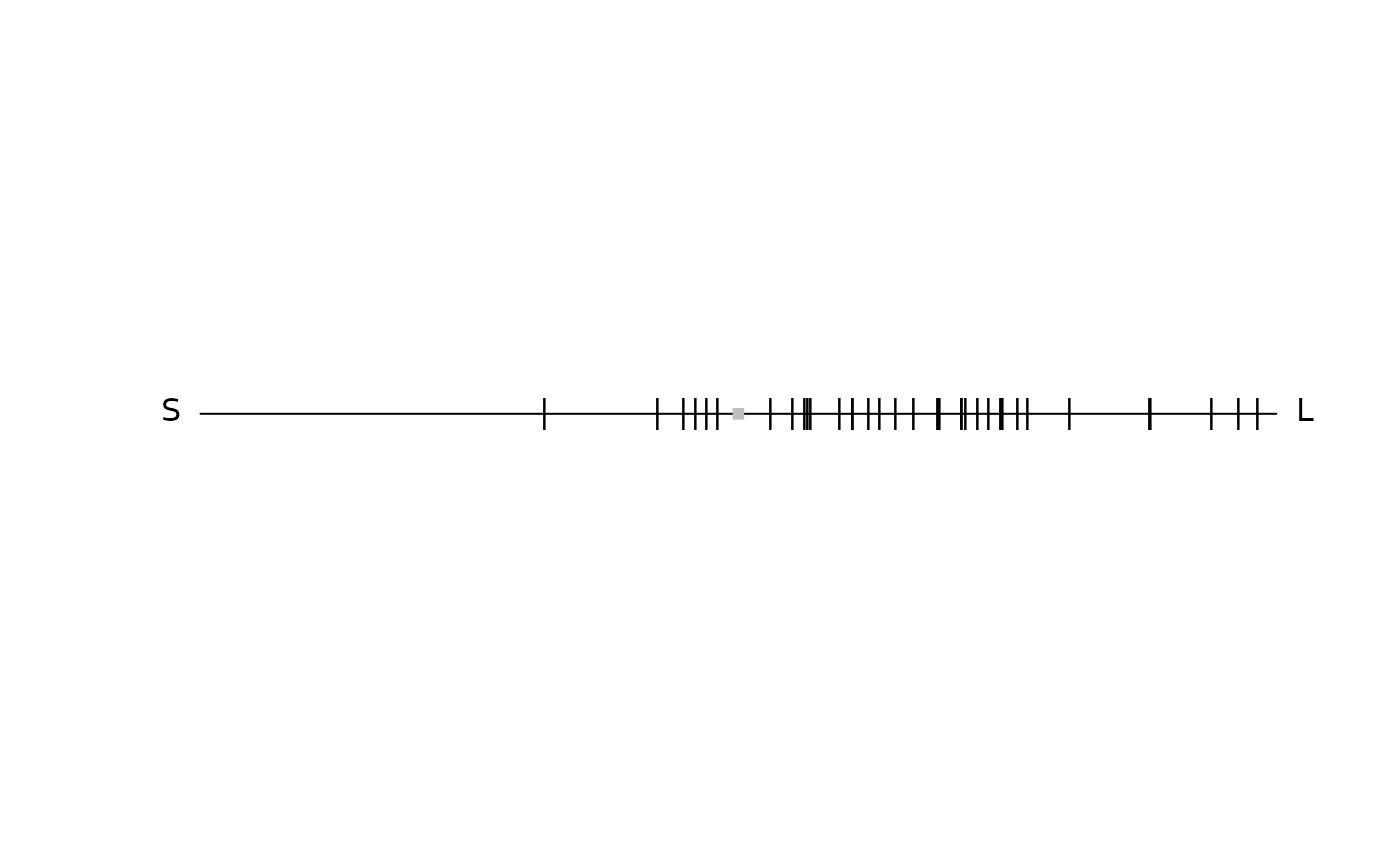Produces a dichromat segment plot.

diplot(
didata,
labels = TRUE,
achro = TRUE,
achrocol = "grey",
achrosize = 0.8,
labels.cex = 1,
out.lwd = 1,
out.lcol = "black",
out.lty = 1,
square = TRUE,
margins = NULL,
...
)

## Arguments

didata

(required) a data frame, possibly a result from the colspace() or dispace() function, containing values for the 'x' coordinates as a column (labeled as such).

labels

logical. Should the name of each cone be printed next to the corresponding vertex?

achro

should a point be plotted at the origin (defaults to TRUE)?

achrocol

color of the point at the origin achro = TRUE (defaults to 'grey').

achrosize

size of the point at the origin when achro = TRUE (defaults to 0.8).

labels.cex

size of the arrow labels.

out.lwd

graphical parameters for the plot outline.

out.lcol

graphical parameters for the plot outline.

out.lty

graphical parameters for the plot outline.

square

logical. Should the aspect ratio of the plot be held to 1:1? (defaults to TRUE).

margins

Deprecated. Please use the standard par() method for custom margins.

...

additional graphical options. See par().

## Author

Thomas White thomas.white026@gmail.com

## Examples

data(flowers)
vis.flowers <- vismodel(flowers, visual = "canis")
di.flowers <- colspace(vis.flowers, space = "di")
plot(di.flowers)